SNAP Library 6.0, Developer Reference  2020-12-09 16:24:20 SNAP, a general purpose, high performance system for analysis and manipulation of large networks
TRowIteratorWithRemove Class Reference

Iterator class for TTable rows, that allows logical row removal while iterating. More...

`#include <table.h>`

Collaboration diagram for TRowIteratorWithRemove: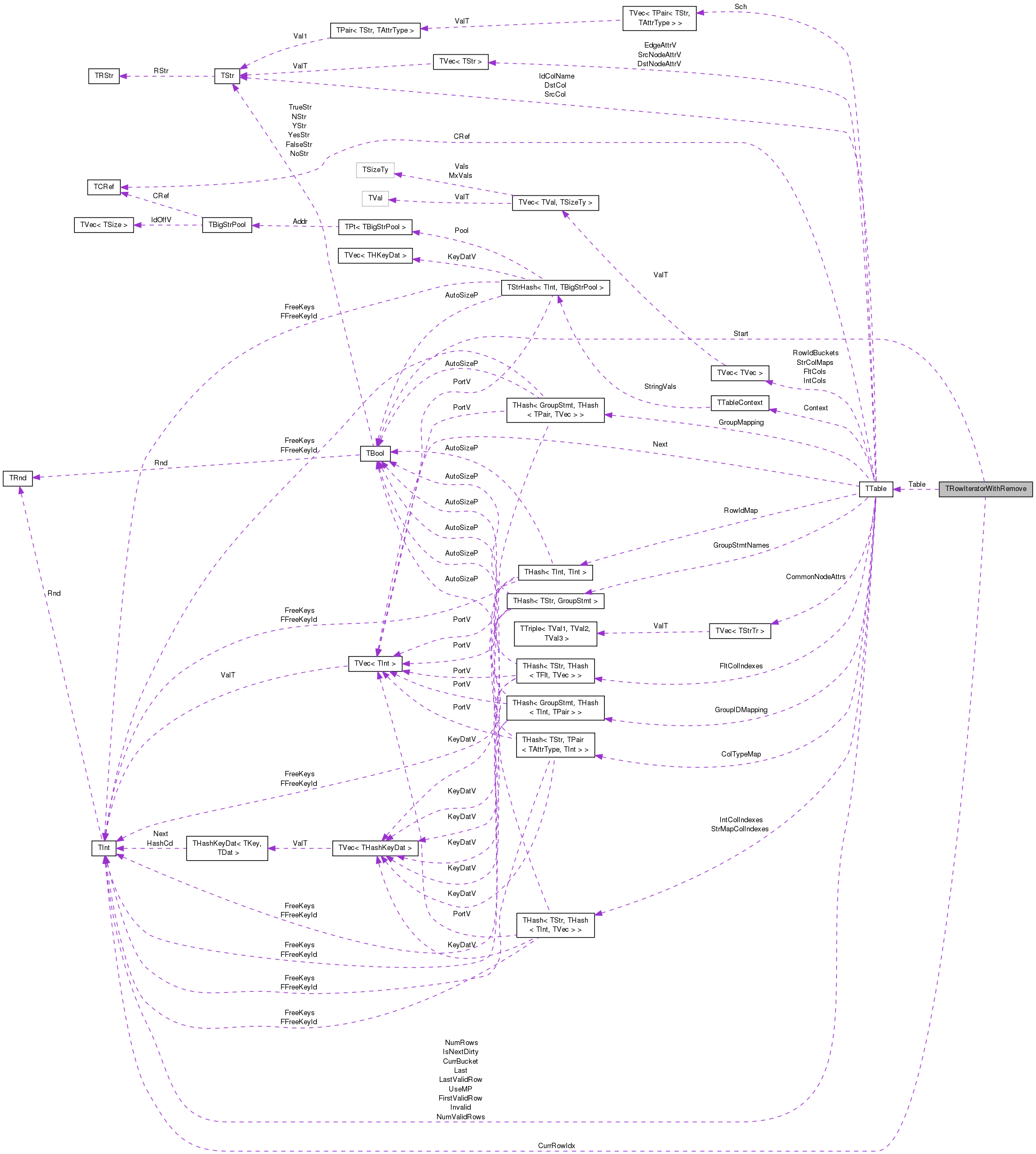[legend]

## Public Member Functions

TRowIteratorWithRemove ()
Default constructor. More...

TRowIteratorWithRemove (TInt RowIdx, TTable *TablePtr)
Constructs iterator pointing to given row. More...

TRowIteratorWithRemove (TInt RowIdx, TTable *TablePtr, TBool IsStart)
Constructs iterator pointing to given row. More...

TRowIteratorWithRemove (const TRowIteratorWithRemove &RowI)
Copy constructor. More...

TRowIteratorWithRemoveoperator++ (int)
Increments the iterator. More...

TRowIteratorWithRemoveNext ()
Increments the iterator (For Python compatibility). More...

bool operator< (const TRowIteratorWithRemove &RowI) const
Checks if this iterator points to a row that is before the one pointed by `RowI`. More...

bool operator== (const TRowIteratorWithRemove &RowI) const
Checks if this iterator points to the same row pointed by `RowI`. More...

TInt GetRowIdx () const
Gets physical index of current row. More...

TInt GetNextRowIdx () const
Gets physical index of next row. More...

TInt GetNextIntAttr (TInt ColIdx) const
Returns value of integer attribute specified by integer column index for next row. More...

TFlt GetNextFltAttr (TInt ColIdx) const
Returns value of float attribute specified by float column index for next row. More...

TStr GetNextStrAttr (TInt ColIdx) const
Returns value of string attribute specified by string column index for next row. More...

TInt GetNextIntAttr (const TStr &Col) const
Returns value of integer attribute specified by attribute name for next row. More...

TFlt GetNextFltAttr (const TStr &Col) const
Returns value of float attribute specified by attribute name for next row. More...

TStr GetNextStrAttr (const TStr &Col) const
Returns value of string attribute specified by attribute name for next row. More...

TBool IsFirst () const
Checks whether iterator points to first valid row of the table. More...

void RemoveNext ()
Removes next row. More...

TBool CompareAtomicConst (TInt ColIdx, const TPrimitive &Val, TPredComp Cmp)
Compares value in column `ColIdx` with given primitive `Val`. More...

## Private Attributes

TInt CurrRowIdx
Physical row index of current row pointer by iterator. More...

TTableTable
Reference to table containing this row. More...

TBool Start
A flag indicating whether the current row in the first valid row of the table. More...

## Detailed Description

Iterator class for TTable rows, that allows logical row removal while iterating.

Definition at line 374 of file table.h.

## Constructor & Destructor Documentation

 TRowIteratorWithRemove::TRowIteratorWithRemove ( )
inline

Default constructor.

Definition at line 380 of file table.h.

380 : CurrRowIdx(0), Table(NULL), Start(true) {}
TBool Start
A flag indicating whether the current row in the first valid row of the table.
Definition: table.h:377
TTable * Table
Reference to table containing this row.
Definition: table.h:376
TInt CurrRowIdx
Physical row index of current row pointer by iterator.
Definition: table.h:375
 TRowIteratorWithRemove::TRowIteratorWithRemove ( TInt RowIdx, TTable * TablePtr )

Constructs iterator pointing to given row.

Definition at line 215 of file table.cpp.

215  :
216  CurrRowIdx(RowIdx), Table(TablePtr), Start(RowIdx == TablePtr->FirstValidRow) {}
TInt FirstValidRow
Physical index of first valid row.
Definition: table.h:553
TBool Start
A flag indicating whether the current row in the first valid row of the table.
Definition: table.h:377
TTable * Table
Reference to table containing this row.
Definition: table.h:376
TInt CurrRowIdx
Physical row index of current row pointer by iterator.
Definition: table.h:375
 TRowIteratorWithRemove::TRowIteratorWithRemove ( TInt RowIdx, TTable * TablePtr, TBool IsStart )
inline

Constructs iterator pointing to given row.

Definition at line 384 of file table.h.

384  : CurrRowIdx(RowIdx),
385  Table(TablePtr), Start(IsStart) {}
TBool Start
A flag indicating whether the current row in the first valid row of the table.
Definition: table.h:377
TTable * Table
Reference to table containing this row.
Definition: table.h:376
TInt CurrRowIdx
Physical row index of current row pointer by iterator.
Definition: table.h:375
 TRowIteratorWithRemove::TRowIteratorWithRemove ( const TRowIteratorWithRemove & RowI )
inline

Copy constructor.

Definition at line 387 of file table.h.

387  : CurrRowIdx(RowI.CurrRowIdx),
388  Table(RowI.Table), Start(RowI.Start) {}
TBool Start
A flag indicating whether the current row in the first valid row of the table.
Definition: table.h:377
TTable * Table
Reference to table containing this row.
Definition: table.h:376
TInt CurrRowIdx
Physical row index of current row pointer by iterator.
Definition: table.h:375

## Member Function Documentation

 TBool TRowIteratorWithRemove::CompareAtomicConst ( TInt ColIdx, const TPrimitive & Val, TPredComp Cmp )

Compares value in column `ColIdx` with given primitive `Val`.

Definition at line 282 of file table.cpp.

Referenced by TTable::SelectAtomicConst().

282  {
283  TBool Result;
284  switch (Val.GetType()) {
285  case atInt:
286  Result = TPredicate::EvalAtom(GetNextIntAttr(ColIdx), Val.GetInt(), Cmp);
287  break;
288  case atFlt:
289  Result = TPredicate::EvalAtom(GetNextFltAttr(ColIdx), Val.GetFlt(), Cmp);
290  break;
291  case atStr:
292  Result = TPredicate::EvalStrAtom(GetNextStrAttr(ColIdx), Val.GetStr(), Cmp);
293  break;
294  default:
295  Result = TBool(false);
296  }
297  return Result;
298 }
TFlt GetNextFltAttr(TInt ColIdx) const
Returns value of float attribute specified by float column index for next row.
Definition: table.cpp:252
TStr GetNextStrAttr(TInt ColIdx) const
Returns value of string attribute specified by string column index for next row.
Definition: table.cpp:256
static TBool EvalStrAtom(const TStr &Val1, const TStr &Val2, TPredComp Cmp)
Compare atomic string values Val1 and Val2 using predicate Cmp.
Definition: table.h:123
Definition: gbase.h:23
TStr GetStr() const
Definition: table.h:228
TInt GetInt() const
Definition: table.h:226
TAttrType GetType() const
Definition: table.h:229
Definition: gbase.h:23
static TBool EvalAtom(T Val1, T Val2, TPredComp Cmp)
Compare atomic values Val1 and Val2 using predicate Cmp.
Definition: table.h:110
bool Cmp(const int &RelOp, const TRec &Rec1, const TRec &Rec2)
Definition: bd.h:426
Definition: gbase.h:23
TInt GetNextIntAttr(TInt ColIdx) const
Returns value of integer attribute specified by integer column index for next row.
Definition: table.cpp:248
Definition: dt.h:974
TFlt GetFlt() const
Definition: table.h:227

Here is the call graph for this function: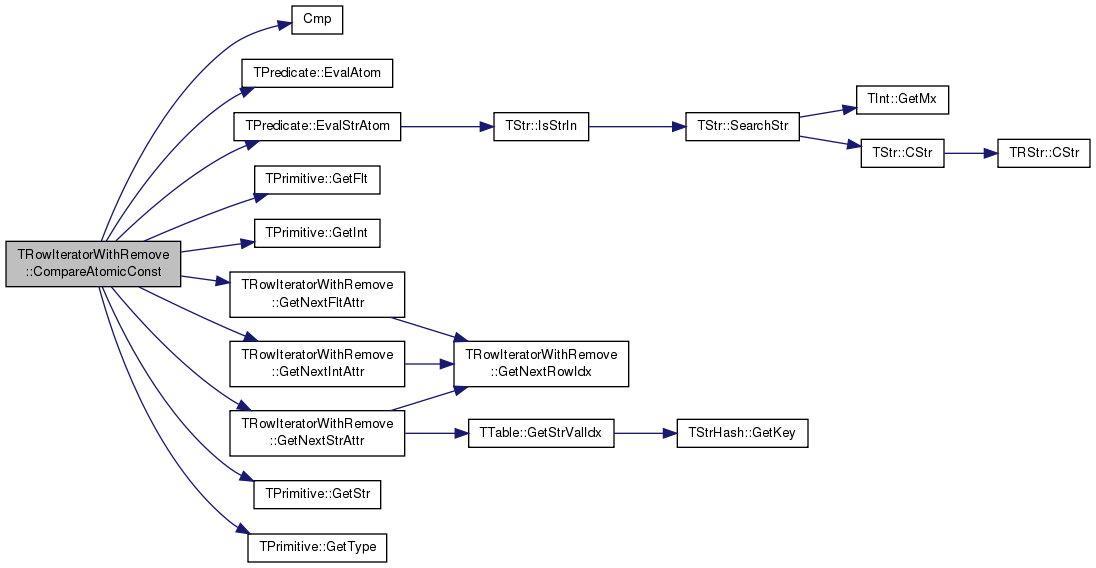Here is the caller graph for this function: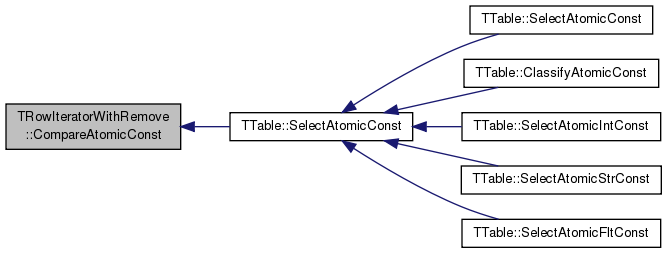TFlt TRowIteratorWithRemove::GetNextFltAttr ( TInt ColIdx ) const

Returns value of float attribute specified by float column index for next row.

Definition at line 252 of file table.cpp.

References TTable::FltCols, GetNextRowIdx(), and Table.

Referenced by CompareAtomicConst(), TTable::Select(), and TTable::SelectAtomic().

252  {
253  return Table->FltCols[ColIdx][GetNextRowIdx()];
254 }
TInt GetNextRowIdx() const
Gets physical index of next row.
Definition: table.cpp:243
TVec< TFltV > FltCols
Data columns of floating point attributes.
Definition: table.h:559
TTable * Table
Reference to table containing this row.
Definition: table.h:376

Here is the call graph for this function: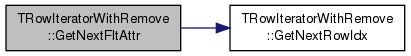Here is the caller graph for this function: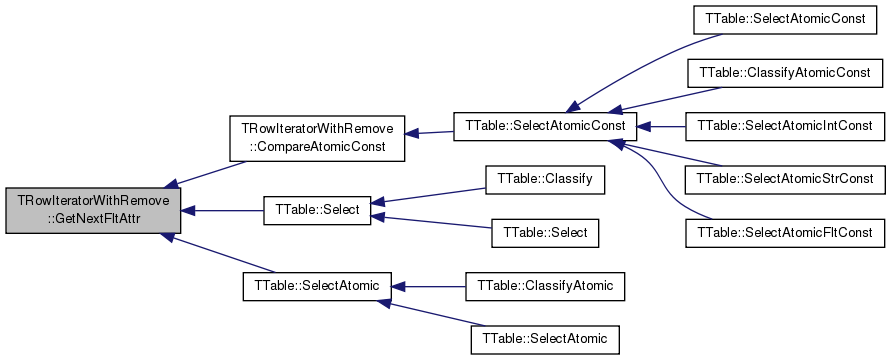TFlt TRowIteratorWithRemove::GetNextFltAttr ( const TStr & Col ) const

Returns value of float attribute specified by attribute name for next row.

Definition at line 265 of file table.cpp.

References TTable::FltCols, TTable::GetColIdx(), GetNextRowIdx(), and Table.

265  {
266  TInt ColIdx = Table->GetColIdx(Col);
267  return Table->FltCols[ColIdx][GetNextRowIdx()];
268 }
TInt GetColIdx(const TStr &ColName) const
Gets index of column ColName among columns of the same type in the schema.
Definition: table.h:1013
TInt GetNextRowIdx() const
Gets physical index of next row.
Definition: table.cpp:243
Definition: dt.h:1137
TVec< TFltV > FltCols
Data columns of floating point attributes.
Definition: table.h:559
TTable * Table
Reference to table containing this row.
Definition: table.h:376

Here is the call graph for this function: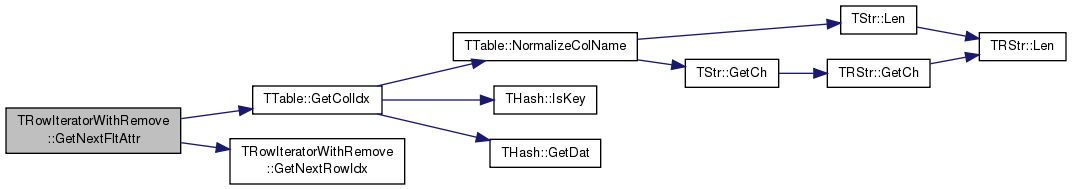TInt TRowIteratorWithRemove::GetNextIntAttr ( TInt ColIdx ) const

Returns value of integer attribute specified by integer column index for next row.

Definition at line 248 of file table.cpp.

References GetNextRowIdx(), TTable::IntCols, and Table.

Referenced by CompareAtomicConst(), TTable::Select(), and TTable::SelectAtomic().

248  {
249  return Table->IntCols[ColIdx][GetNextRowIdx()];
250 }
TVec< TIntV > IntCols
Next[i] is the successor of row i. Table iterators follow the order dictated by Next ...
Definition: table.h:558
TInt GetNextRowIdx() const
Gets physical index of next row.
Definition: table.cpp:243
TTable * Table
Reference to table containing this row.
Definition: table.h:376

Here is the call graph for this function: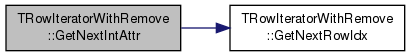Here is the caller graph for this function: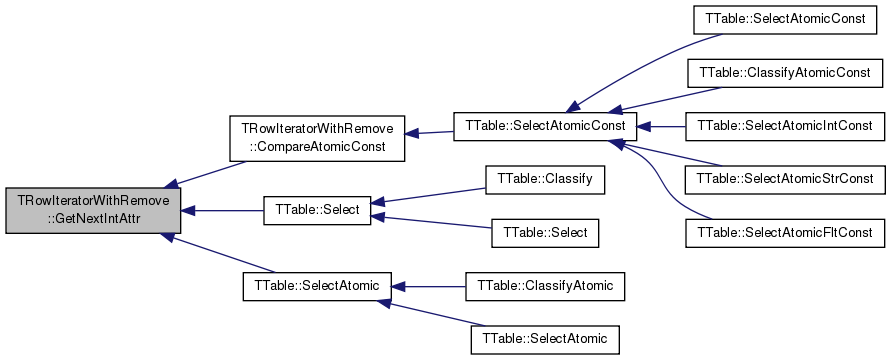TInt TRowIteratorWithRemove::GetNextIntAttr ( const TStr & Col ) const

Returns value of integer attribute specified by attribute name for next row.

Definition at line 260 of file table.cpp.

References TTable::GetColIdx(), GetNextRowIdx(), TTable::IntCols, and Table.

260  {
261  TInt ColIdx = Table->GetColIdx(Col);
262  return Table->IntCols[ColIdx][GetNextRowIdx()];
263 }
TInt GetColIdx(const TStr &ColName) const
Gets index of column ColName among columns of the same type in the schema.
Definition: table.h:1013
TVec< TIntV > IntCols
Next[i] is the successor of row i. Table iterators follow the order dictated by Next ...
Definition: table.h:558
TInt GetNextRowIdx() const
Gets physical index of next row.
Definition: table.cpp:243
Definition: dt.h:1137
TTable * Table
Reference to table containing this row.
Definition: table.h:376

Here is the call graph for this function: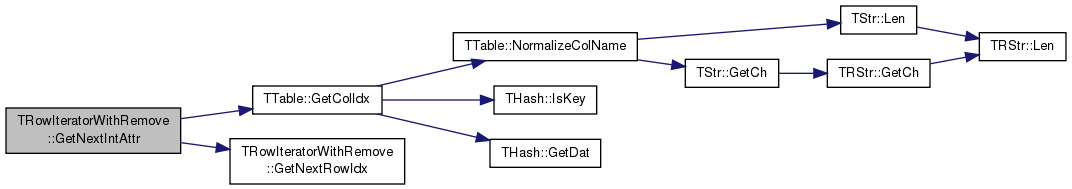TInt TRowIteratorWithRemove::GetNextRowIdx ( ) const

Gets physical index of next row.

Definition at line 243 of file table.cpp.

References CurrRowIdx, TTable::FirstValidRow, TTable::Next, Start, and Table.

243  {
244  return (Start ? Table->FirstValidRow : Table->Next[CurrRowIdx]);
245 }
TInt FirstValidRow
Physical index of first valid row.
Definition: table.h:553
TBool Start
A flag indicating whether the current row in the first valid row of the table.
Definition: table.h:377
TIntV Next
A vector describing the logical order of the rows.
Definition: table.h:555
TTable * Table
Reference to table containing this row.
Definition: table.h:376
TInt CurrRowIdx
Physical row index of current row pointer by iterator.
Definition: table.h:375

Here is the caller graph for this function: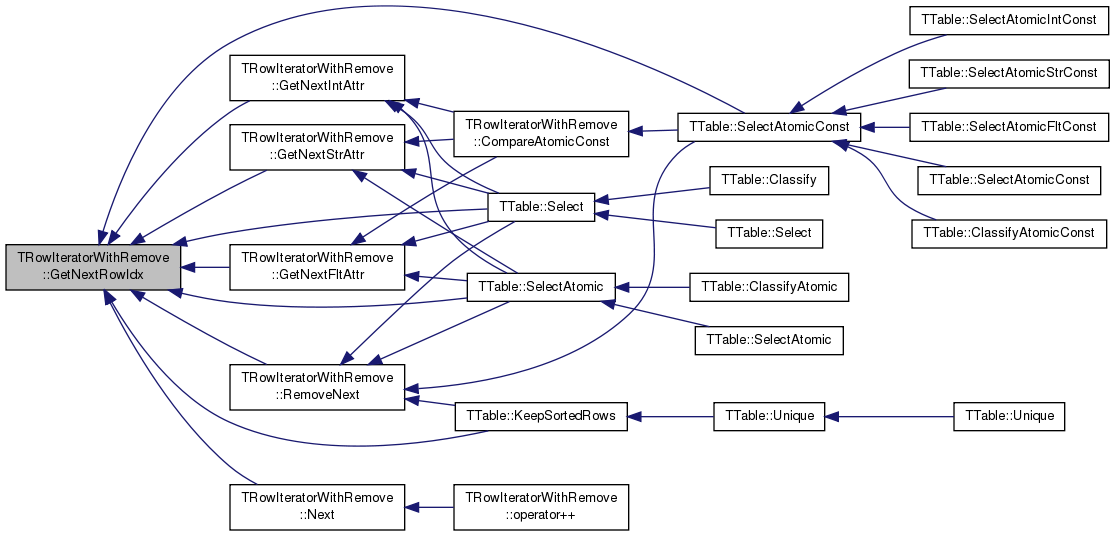TStr TRowIteratorWithRemove::GetNextStrAttr ( TInt ColIdx ) const

Returns value of string attribute specified by string column index for next row.

Definition at line 256 of file table.cpp.

References GetNextRowIdx(), TTable::GetStrValIdx(), and Table.

Referenced by CompareAtomicConst(), TTable::Select(), and TTable::SelectAtomic().

256  {
257  return Table->GetStrValIdx(ColIdx, GetNextRowIdx());
258 }
TStr GetStrValIdx(TInt ColIdx, TInt RowIdx) const
Gets the value in column with id ColIdx at row RowIdx.
Definition: table.h:626
TInt GetNextRowIdx() const
Gets physical index of next row.
Definition: table.cpp:243
TTable * Table
Reference to table containing this row.
Definition: table.h:376

Here is the call graph for this function:Here is the caller graph for this function: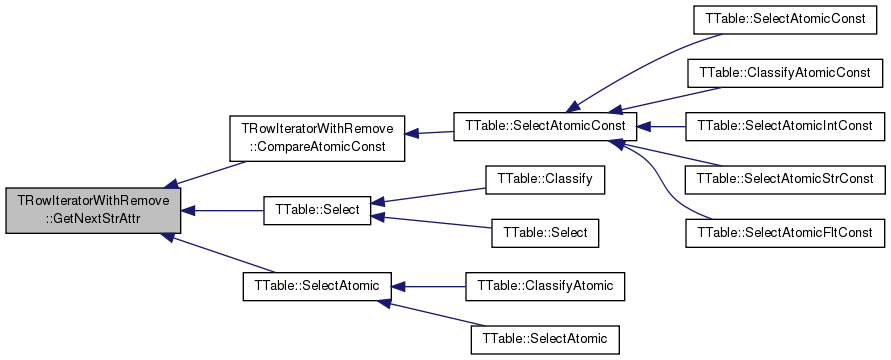TStr TRowIteratorWithRemove::GetNextStrAttr ( const TStr & Col ) const

Returns value of string attribute specified by attribute name for next row.

Definition at line 270 of file table.cpp.

References GetNextRowIdx(), TTable::GetStrVal(), and Table.

270  {
271  return Table->GetStrVal(Col, GetNextRowIdx());
272 }
TInt GetNextRowIdx() const
Gets physical index of next row.
Definition: table.cpp:243
TTable * Table
Reference to table containing this row.
Definition: table.h:376
TStr GetStrVal(const TStr &ColName, const TInt &RowIdx) const
Gets the value of string attribute ColName at row RowIdx.
Definition: table.h:1028

Here is the call graph for this function: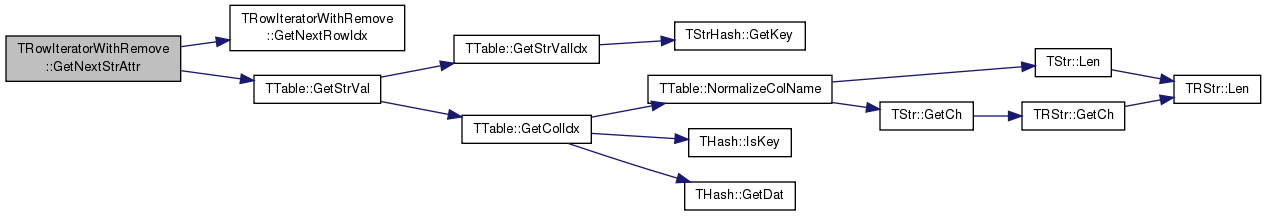TInt TRowIteratorWithRemove::GetRowIdx ( ) const

Gets physical index of current row.

Definition at line 239 of file table.cpp.

References CurrRowIdx.

239  {
240  return CurrRowIdx;
241 }
TInt CurrRowIdx
Physical row index of current row pointer by iterator.
Definition: table.h:375
 TBool TRowIteratorWithRemove::IsFirst ( ) const

Checks whether iterator points to first valid row of the table.

Definition at line 274 of file table.cpp.

References CurrRowIdx, TTable::FirstValidRow, and Table.

274  {
275  return CurrRowIdx == Table->FirstValidRow;
276 }
TInt FirstValidRow
Physical index of first valid row.
Definition: table.h:553
TTable * Table
Reference to table containing this row.
Definition: table.h:376
TInt CurrRowIdx
Physical row index of current row pointer by iterator.
Definition: table.h:375
 TRowIteratorWithRemove & TRowIteratorWithRemove::Next ( )

Increments the iterator (For Python compatibility).

Definition at line 222 of file table.cpp.

References Assert, CurrRowIdx, GetNextRowIdx(), and Start.

Referenced by operator++().

222  {
224  Start = false;
226  return *this;
227 }
TBool Start
A flag indicating whether the current row in the first valid row of the table.
Definition: table.h:377
TInt GetNextRowIdx() const
Gets physical index of next row.
Definition: table.cpp:243
#define Assert(Cond)
Definition: bd.h:251
static const TInt Invalid
Special value for Next vector entry - logically removed row.
Definition: table.h:487
TInt CurrRowIdx
Physical row index of current row pointer by iterator.
Definition: table.h:375

Here is the call graph for this function: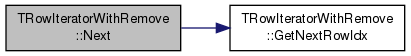Here is the caller graph for this function: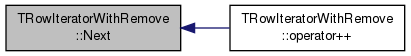TRowIteratorWithRemove & TRowIteratorWithRemove::operator++ ( int )

Increments the iterator.

Definition at line 218 of file table.cpp.

References Next().

218  {
219  return this->Next();
220 }
TRowIteratorWithRemove & Next()
Increments the iterator (For Python compatibility).
Definition: table.cpp:222

Here is the call graph for this function: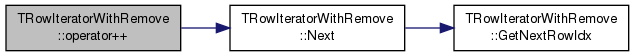bool TRowIteratorWithRemove::operator< ( const TRowIteratorWithRemove & RowI ) const

Checks if this iterator points to a row that is before the one pointed by `RowI`.

Definition at line 229 of file table.cpp.

References CurrRowIdx.

229  {
230  if (CurrRowIdx == TTable::Last) { return false; }
231  if (RowI.CurrRowIdx == TTable::Last) { return true; }
232  return CurrRowIdx < RowI.CurrRowIdx;
233 }
static const TInt Last
Special value for Next vector entry - last row in table.
Definition: table.h:486
TInt CurrRowIdx
Physical row index of current row pointer by iterator.
Definition: table.h:375
 bool TRowIteratorWithRemove::operator== ( const TRowIteratorWithRemove & RowI ) const

Checks if this iterator points to the same row pointed by `RowI`.

Definition at line 235 of file table.cpp.

References CurrRowIdx.

235  {
236  return CurrRowIdx == RowI.CurrRowIdx;
237 }
TInt CurrRowIdx
Physical row index of current row pointer by iterator.
Definition: table.h:375
 void TRowIteratorWithRemove::RemoveNext ( )

Removes next row.

Definition at line 278 of file table.cpp.

References CurrRowIdx, GetNextRowIdx(), TTable::RemoveRow(), and Table.

278  {
280 }
void RemoveRow(TInt RowIdx, TInt PrevRowIdx)
Removes row with id RowIdx.
Definition: table.cpp:1135
TInt GetNextRowIdx() const
Gets physical index of next row.
Definition: table.cpp:243
TTable * Table
Reference to table containing this row.
Definition: table.h:376
TInt CurrRowIdx
Physical row index of current row pointer by iterator.
Definition: table.h:375

Here is the call graph for this function: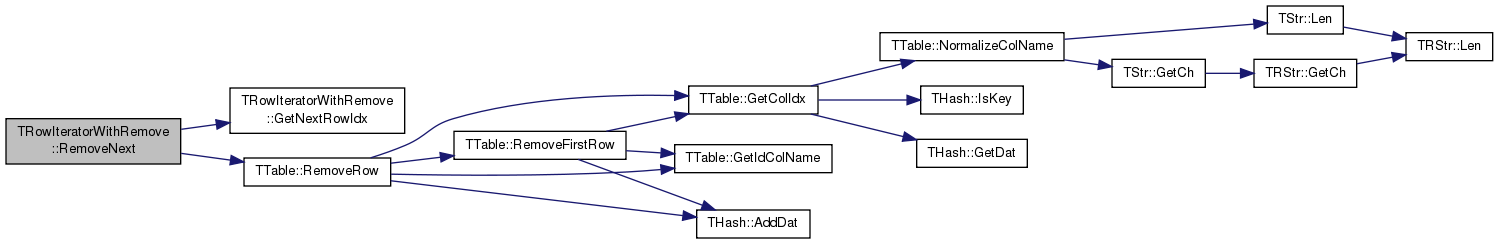Here is the caller graph for this function: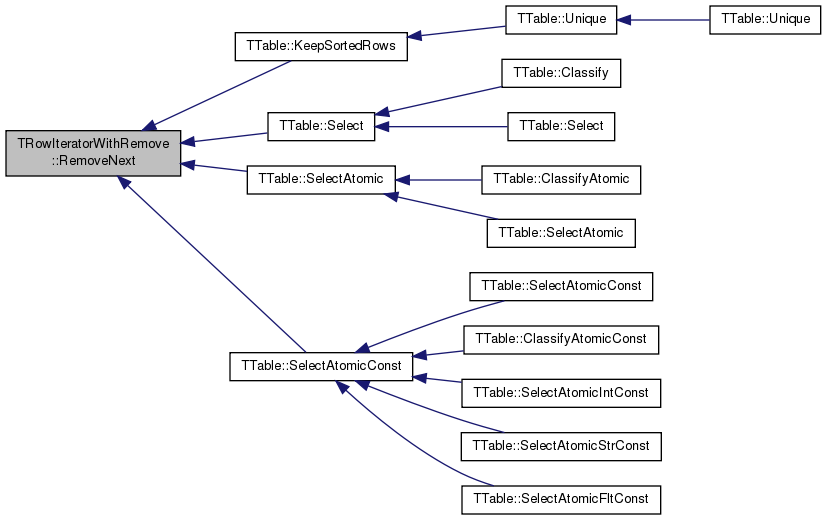## Member Data Documentation

 TInt TRowIteratorWithRemove::CurrRowIdx
private

Physical row index of current row pointer by iterator.

Definition at line 375 of file table.h.

Referenced by GetNextRowIdx(), GetRowIdx(), IsFirst(), Next(), operator<(), operator==(), and RemoveNext().

 TBool TRowIteratorWithRemove::Start
private

A flag indicating whether the current row in the first valid row of the table.

Definition at line 377 of file table.h.

Referenced by GetNextRowIdx(), and Next().

 TTable* TRowIteratorWithRemove::Table
private

Reference to table containing this row.

Definition at line 376 of file table.h.

Referenced by GetNextFltAttr(), GetNextIntAttr(), GetNextRowIdx(), GetNextStrAttr(), IsFirst(), and RemoveNext().

The documentation for this class was generated from the following files: